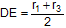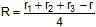In the figure below, ABC is a triangle inscribed in a circle of center O (circumcenter), I is the incenter and E1, E2, E3 are the excenters  relatives to the sides BC, AC, and AB respectively. Diameter DF of center O is perpendicular to AC at point E. If R, r, r1, r2, r3 are the circumradius, inradius and exradii respectively, (1) prove that, (2) prove that, and (3) prove that.